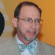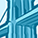cancel
Showing results for
Did you mean:New Contributor

## Why do internal energy/heat and enthalphy differ? What is the physical significance of the PV term for enthalphy of an ideal gas?

Hello all! I have a quick and stupid thermodynamics question.

The enthalpy of an ideal gas is h = u + PV. I understand that the specific internal energy, u, is the sum of all rotational, vibrational, translational, electronic/nuclear bonding, and lattice (ignored for ideal gasses, I presume) energies of the substance per unit mass. I further understand that w + q =\Delta u for specific transferred  work and heat w and q, implying that \delta w + \delta q = du (forgive my lowercase deltas in place of a proper inexact differential symbol). Finally, I understand that since h = u + PV and PV = N * Rhat * T, for a constant amount of ideal gas, PV is solely a function of temperature. It can be demonstrated that for an ideal gas, u is also a function solely of temperature, so that means his as well.

So what's the difference between u and h? Clearly, it's that PV term, but what does that physically represent? I don't grok it.

All incoming energy, whether mechanical or thermal, goes into a change of u--that makes sense. Add energy to a gas, it's going to vibrate/translate/rotate more & maybe change its bonding state. Say you adiabatically compress your gas. You do work on it and u and T rise, but PV stays constant. Adiabatically unconfine the gas and the same thing occurs the other way. But h still varies because u does, it's not conserved over adiabatic actions.

So the "energy" of the PV term represents something somehow separate to internal energy, inherent to the system, invariate to mechanical work done on it... unless that work ends up raising the temperature? What is this term physically represent? Where does it exist? Why bother with the enthalpy concept at all? I don't get it & I know I'm missing something fundamental.

4 RepliesContributor III

## Re: Why do internal energy/heat and enthalphy differ? What is the physical significance of the PV term for enthalphy of an ideal gas?

Dear Anton,

It is important in the sciences to always remember that we are just trying to make sense of observations. Thus, sometimes mathematical derivations come from different observed terms. It is always interesting to see how the mathematical manipulations and comparisons can reveal other things that might not have been noticed by the direct observations (or bias) of the experiments.

Gas phase interactions are different from the other phases,with that unique PV=nRT relationship. What you are seeing is the expression of the combination of the “internal” or intrinsic energy factors with the “external” or environmental factors. “PV” (and T) are external conditions, all related to the energy of the system and the atoms in it. In the Gas Laws we are relating the observed energy of the system (PVT) to the number of molecules ‘n’ with the derived constant ‘R’. Enthalpy is the heat energy in a system, not all of which is accessible. That is the specific internal energy ‘u’. The PV term expresses the accessible system energy as observed and related in the Gas Laws. COMBINED they express the total enthalpy of the molecule. Because we are expressing the energy in terms of heat, the temperature becomes another label for the heat energy in the equations.

Again, mathematical manipulations are always fun and internally consistent when done properly. Sometimes they can even uncover possible physical relationships that we didn’t originally observe. But in the end the primary importance of any mathematical expression is to model a real observation (experiment) accurately to allow us to predict future outcomes from different conditions. Keep in mind also where we get our symbols from. The ‘PV’ is not a ‘term’, but the expression of an observed Pressure and Volume in the system. Pressure, volume, and temperature (PVT) can all vary independently as well as being connected as expressed in the Gas Laws when part of a closed system.

Best regards,

StevenNew Contributor

## Re: Why do internal energy/heat and enthalphy differ? What is the physical significance of the PV term for enthalphy of an ideal gas?

Anton asked a great question implying that he is good at thinking fundamental things through. My understanding is like this: a) for solid or liquid systems that are not reactive, PV is minimal, H is equivalent to U (or Q when W=0); for a closed system of a gas, the PV term (equals nRT)  is no more negligible because a gas tends to expand significantly when T changes. This PV or nRT is part of H on top of U. b) for reactive systems, H represents reactivity or heat of reactions including newly settled inherent U plus residual heat (to be absorbed - endothermic or to be released -exothermic); in the case of a closed gas system, this portion of residual heat inevitably drops or increases the T and it leads to a change in PV accordingly. Again, for solid and liquid systems, the PV part remains negligible since they are not compressible or expandable like gases at all.New Contributor

## Re: Why do internal energy/heat and enthalphy differ? What is the physical significance of the PV term for enthalphy of an ideal gas?

Dear Anton,

Excellent question! I would like to elaborate a little on the way change in internal energy and change in enthalpy relate to commonly done experiments and think this may help clarify the pV term.

Constant volume calorimetry, freqently called 'bomb' calorimetry, measures change in internal energy.  There is no (or negligible) change in volume of the system so no work is done on or by the system, w = -p(delta V) = 0 so delta U = q.  Constant pressure calorimetry, frequently done in a thermal cup at atmospheric pressure which remains constant through the experiment is a method of measuring enthalpy changes.   At constant pressure, the volume of the system changes based on the reaction taking place so work is done, delta H = q = delta U + p(delta V) = delta U + (delta n)RT where delta n is the change in moles of gas phase species with the reaction. The p(delta V) = (delta n)RT term may be small but non negligible if moles of gas phase reactants and products are different.  Note that q measured under these different circumstances, constant V or constant p, are not the same.

Enthalpy is an important state function and enthalpy changes are tabulated more frequently than internal energy changes because measurement of q at constant pressure entails easier experiments with less expensive experimental set ups.  Thank you for posting this interesting and important question.New Contributor

## Re: Why do internal energy/heat and enthalphy differ? What is the physical significance of the PV term for enthalphy of an ideal gas?

I consider the PV term to represent to translational energy of the gas entities (atoms or molecules).  This is the physical significance of the PV term.  Let's consider argon, a monatomic gas with no rotational or vibrational components for energy, only translation.   The movement of the atoms leads to collisions with the wall, producing pressure on the wall.

If we let a fixed weight of argon gas to flow into a soap bubble the volume of the bubble will increase until the pressure from collisions inside the bubble equals the pressure outside (plus any small tension from the soap bubble's film).  if we cool the system (without changing the outside pressure) the translational energy, as well as the volume of the bubble, will decrease linearly with the Kelvin (absolute) temperature.